$$\newcommand{\vecs}{\overset { \rightharpoonup} {\mathbf{#1}} }$$ $$\newcommand{\vecd}{\overset{-\!-\!\rightharpoonup}{\vphantom{a}\smash {#1}}}$$$$\newcommand{\id}{\mathrm{id}}$$ $$\newcommand{\Span}{\mathrm{span}}$$ $$\newcommand{\kernel}{\mathrm{null}\,}$$ $$\newcommand{\range}{\mathrm{range}\,}$$ $$\newcommand{\RealPart}{\mathrm{Re}}$$ $$\newcommand{\ImaginaryPart}{\mathrm{Im}}$$ $$\newcommand{\Argument}{\mathrm{Arg}}$$ $$\newcommand{\norm}{\| #1 \|}$$ $$\newcommand{\inner}{\langle #1, #2 \rangle}$$ $$\newcommand{\Span}{\mathrm{span}}$$ $$\newcommand{\id}{\mathrm{id}}$$ $$\newcommand{\Span}{\mathrm{span}}$$ $$\newcommand{\kernel}{\mathrm{null}\,}$$ $$\newcommand{\range}{\mathrm{range}\,}$$ $$\newcommand{\RealPart}{\mathrm{Re}}$$ $$\newcommand{\ImaginaryPart}{\mathrm{Im}}$$ $$\newcommand{\Argument}{\mathrm{Arg}}$$ $$\newcommand{\norm}{\| #1 \|}$$ $$\newcommand{\inner}{\langle #1, #2 \rangle}$$ $$\newcommand{\Span}{\mathrm{span}}$$

We turn now to discuss a few specific addition reactions of the carbonyl groups of aldehydes and ketones. We shall not attempt to provide an extensive catalog of reactions, but will try to emphasize the principles involved with especially important reactions that are useful in synthesis.

Grignard reagents, organolithium compounds, and the like generally add to aldehydes and ketones rapidly and irreversibly, but the same is not true of many other reagents; their addition reactions may require acidic or basic catalysts; the adducts may be formed reversibly and with relatively unfavorable equilibrium constants. Also, the initial adducts may be unstable and react further by elimination. (We recommend that you review Section 15-4E to see examples of these points.) To organize this very large number of addition reactions, we have arranged the reactions according to the nucleophile that adds to the carbonyl carbon. The types of nucleophiles considered here form $$\ce{C-C}$$, $$\ce{C-O}$$, $$\ce{C-N}$$, $$\ce{C}$$-halogen, $$\ce{C-S}$$, and $$\ce{C-H}$$ bonds. A summary is given in Table 16-4.

Table 16-4: Addition Reactions of Aldehydes and Ketones### Cyanohydrin Formation

Hydrogen cyanide adds to many aldehydes and ketones to give hydroxylnitriles, usually called "cyanohydrins":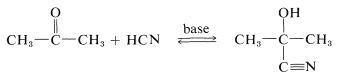The products are useful in synthesis - for example, in the preparation of cyanoalkenes and hydroxy acids:An important feature of cyanohydrin formation is that it requires a basic catalyst. In the absence of base, the reaction does not proceed, or is at best very slow. In principle, the basic catalyst may activate either the carbonyl group or hydrogen cyanide. With hydroxide ion as the base, one reaction to be expected is a reversible addition of hydroxide to the carbonyl group:However, such addition is not likely to facilitate formation of cyanohydrin because it represents a competitive saturation of the carbonyl double bond. Indeed, if the equilibrium constant for this addition were large, an excess of hydroxide ion could inhibit cyanohydrin formation by tying up the ketone as the adduct $$1$$.

Hydrogen cyanide itself has no unshared electron pair on carbon and does not form a carbon-carbon bond to a carbonyl carbon. However, a small amount of a strong base can activate hydrogen cyanide by converting it to cyanide ion, which can function as a carbon nucleophile. A complete sequence for cyanohydrin formation follows:The second step regenerates the cyanide ion. Each step of the reaction is reversible but, with aldehydes and most nonhindered ketones, formation of the cyanohydrin is reasonably favorable. In practical syntheses of cyanohydrins, it is convenient to add a strong acid to a mixture of sodium cyanide and the carbonyl compound, so that hydrogen cyanide is generated in situ. The amount of acid added should be insufficient to consume all the cyanide ion, therefore sufficiently alkaline conditions are maintained for rapid addition.

### Addition of Enolate Anions (See Section 17-3)

There are a number of rather interesting substances for which we can write important dipolar valence-bond structures of the type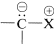. The important factor with these structures is that the negative end of the dipole is carbon with an unshared electron pair. The positive end of the dipole can be several kinds of atoms or groups, the most usual being sulfur, phosphorus, or nitrogen. Some examples (each written here as a single dipolar valence-bond structure) are:The systematic naming of these substances is cumbersome, but they have come to be known as ylides. The genesis of this name may seem obscure, but it is an attempt to reconcile the presence of a $$\ce{C-X}$$ $$\sigma$$ bond, which is covalent and nonpolar as in alkyl derivatives, as well as an ionic bond as in metal halides. Hence, the combination yl-lide.$$^2$$

As we might expect from the dipolar structure, ylides can behave as carbon nucleophiles to form carbon-carbon bonds by addition to the carbonyl groups of aldehydes and ketones:However, the further course of reaction depends on the type of ylide used. In the case of phosphorus ylides, the overall reaction amounts to a very useful synthesis of alkenes by the transfer of oxygen to phosphorus and carbon to carbon, as summarized in Equation 16-1. This is called the Witting reaction:Reactions with sulfur ylides proceed differently. The prodcuts are oxacylcopropanes (oxiranes) - not alkenes. The addition step proceeds as with the phosphorus ylides, but the negatively charged oxygen of the dipolar adduct then displaces the sulfonium group as a neutral sulfide. This is an intramolecular $$S_\text{N}2$$ reaction similar to the formation of oxacyclopropanes from vicinal chloroalcohols (Section 15-11C):As for the nitrogen ylides, a useful reagent of this type is diazomethane, $$\ce{CH_2N_2}$$. Diaxomethane can react with carbonyl compounds in different ways, depending on what happens to the initial adduct $$2$$. Oxacyclopropanes are formed if the nitrogen is simply displaced (as $$\ce{N_2}$$) by oxygen (Path $$a$$, Equation 16-2). Ketones of rearranged carbon framework result if nitrogen is displaced $$as \(\ce{N_2}$$) by $$\ce{R}^\ominus$$ which moves over to the $$\ce{CH_2}$$ group (Path $$b$$, Equation 16-2):Diazoketones, $$\ce{RCOCHN_2}$$, are formed if there is a good leaving group, such as halogen, on the carbonyl (Equation 16-3). Under these circumstances the reactant is an acid halide, not an aldehyde or ketone:## Addition of Oxygen and Sulfur Nucleophiles

### Alcohols, Thiols, Water

We already have discussed additions of alcohols and, by analogy, thiols $$\left( \ce{RSH} \right)$$ to carbonyl compounds (see Section 15-4E). We will not repeat this discussion here except to point out that addition of water to the carbonyl group of an aldehyde is analogous to hemiacetal formation (Section 15-4E) and is catalyzed both by acids and bases: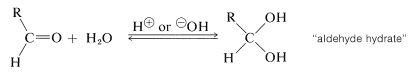The equilibrium for hydrate formation depends both on steric and electrical factors. Methanal is $$99.99\%$$ hydrated in aqueous solution, ethanal is $$58\%$$ hydrated, and 2-propanone is not hydrated significantly. The hydrates seldom can be isolated because they readily revert to the parent aldehyde. The only stable crystalline hydrates known are those having strongly electronegative groups associated with the carbonyl (see Section 15-7).

### Hydrogen Sulfite (Bisulfite) Addition to Carbonyl Compound

Several carbonyl additions have characteristics similar to those of cyanohydrin formation. A typical example is the addition of sodium hydrogen sulfite, which proceeds readily with good conversion in aqueous solution with most aldehydes, methyl ketones, and unhindered cyclic ketones to form a carbon-sulfur bond. No catalyst is required because sulfite is an efficient nucleophilic agent. The addition step evidently involves the sulfite ion - not hydrogen sulfite ion: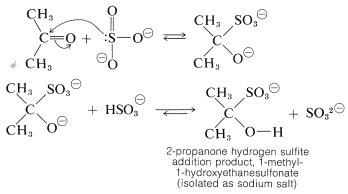The addition products often are nicely crystalline solids that are insoluble in excess concentrated sodium hydrogen sulfite solution. Whether soluble or insoluble, the addition products are useful for separating carbonyl compounds from substances that do not react with sodium hydrogen sulfite.

### Polymerization of Aldehydes

A reaction closely related to acetal formation is the polymerization of aldehydes. Both linear and cyclic polymers are obtained. For example, methanal in water solution polymerizes to a solid ling-chain polymer called paraformaldehyde or "polyoxymethylene":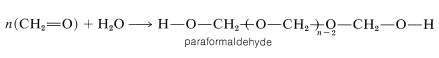This material, when strongly heated, reverts to methanal; it therefore is a convenient source of gaseous methanal. When heated with dilute acid, paraformaldehyde yields the solid trimer, 1,3,5-trioxycyclohexane (mp $$61^\text{o}$$). The cyclic tetramer is also known.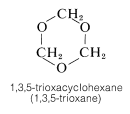Long-chain methanal polymers have become very important as plastics in recent years. The low cost of paraformaldehyde is highly favorable in this connection, but the instability of the material to elevated temperatures and dilute acids precludes its use in plastics. However, the "end-capping" of polyoxymethylene chains through formation of esters or acetals produces a remarkable increase in stability, and such modified polymers have excellent properties as plastics. Delrin (DuPont) is a stabilized methanal polymer with exceptional strengths and ease of molding.

Ethanal (acetaldehyde) polymerizes under the influence of acids to the cyclic trimer, "paraldehyde", and a cyclic tetramer, "metaldehyde". Paraldehyde has been used as a relatively nontoxic sleep-producing drug (hypnotic). Metaldehyde is used as a poison for snails and slugs, "Snarol". Ketones do not appear to form stable polymers like those of aldehydes.## Nitrogen Nucleophiles

### Reactions of $$\ce{RNH_2}$$ Derivatives with Carbonyl Compounds

A wide variety of substances with $$\ce{-NH_2}$$ groups react with aldehydes and ketones by an addition-elimination sequence to givecompounds and water. These reactions usually require acid catalysts:Table 16-5 summarizes several important reactions of this type and the nomenclature of the reactants and products.

Table 16-5: Products from Reactions of Carbonyl Compounds with $$\ce{RNH_2}$$ DerivativesFigure 16-4 shows schematically the type of behavior observed. We can understand the maximum in rate by considering the rates and equilibria involving $$\ce{RNH_2}$$ and the carbonyl compound as well as the rate of dehydration, Equations 16-4 through 16-6.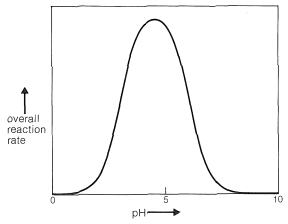Figure 16-4: Schematic variation of the rate of condensation of $$\ce{RNH_2}$$ with a carbonyl compound as a function of pH.

Clearly, if the unshared electron pair on the nitrogen of $$\ce{RNH_2}$$ is combined with a proton, Equation 16-4, it cannot attack the carbonyl carbon to give the aminoalkanol as in Equation 16-5. So at high acid concentration (low pH) we expect the rate and the equilibrium for the overall reaction to be unfavorable. In more dilute acid, the rate picks up because there is more free $$\ce{RNH_2}$$ in solution. Dehydration of the aminoalkanol (Equation 16-6) is acid catalyzed; this reaction normally is fast at pH values smaller than 3-4. Therefore, the slow step at pH $$<$$ 4 is addition of $$\ce{RNH_2}$$ to the carbonyl group as per Equation 16-5. As the pH is increased above 4, the addition becomes progressively faster because less $$\ce{RNH_2}$$ is tied up as $$\ce{RNH_3^+}$$. However, then the dehydration step, Equation 16-6, decreases in rate because it requires an acid catalyst. At pH 6 (remember that going from pH 4 to pH 6 is a 100-fold decrease in $$\ce{H}^\oplus$$ concentration), dehydration is the slow step, and at higher pH values it finally becomes too slow to give a useful overall rate of reaction. This sequence of changes in rate and equilibria has been shown to account precisely for rate vs. pH curves such as in Figure 16-4.

Dehydration of $$\ce{(CH_3)_2CHNR(OH)}$$ to $$\ce{(CH_3)_2C=NR}$$ involves acid catalysis in very much the same way as in acetal formation (Section 15-4E):### Addition of Ammonia to AldehydesThe aldehyde-ammonia adducts usually are not very stable. They readily undergo dehydration and polymerization. 1-Aminoethanol, for example, gives a cyclic trimer composition $$\ce{C_6H_{15}N_3} \cdot 3 \ce{H_2O}$$, mp $$97^\text{o}$$, with structure $$4$$: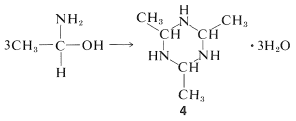Methanal and ammonia react by a different course with the formation of a substance known as "hexamethylenetetramine":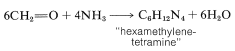The product is a high-melting solid (mp $$> \: 230^\text{o}$$ d.) and its structure has been established by x-ray diffraction (Section 9-3). In fact, it was the first organic substance whose structure was determined in this way. The high melting point is clearly associated with the considerable symmetry and rigidity of the cage structure:The corresponding all-carbon compound, adamantane (Section 12-8), also has a high melting point $$\left( 268^\text{o} \right)$$:Treatment of hexamethylenetetramine with nitric acid gives the high explosive "cyclonite", which often is designated as RDX and was widely used in World War II:Then methanal and ammonia that split off the cage structure during the reaction with nitric acid need not be wasted. In the large-scale manufacture of cyclonite, a combination of nitric acid, ammonium nitrate, and ethanoic anhydride is used, which results in full utilization of the methanal and ammonia:

$\ce{C_6H_{12}N_4} + 4 \ce{HNO_3} + 2 \ce{NH_4NO_3} + 6 \ce{(CH_3CO)_2O} \rightarrow 2 \ce{C_3H_6O_6N_6} + 12 \ce{CH_3CO_2H}$

### Enamines

Secondary amino compounds of the type $$\ce{R_2N-H}$$ add to aldehyde and ketone carbonyl groups in an acid-catalyzed reaction in much the same way as do $$\ce{RNH_2}$$ compounds - with one important difference. The product contains the structural unit $$\ce{C=C-N}$$ rather than $$\ce{C-C=N}$$; and because there is a carbon-carbon double bond, such a substance is called an enamine (alkene $$+$$ amine). An example is:The course of this reaction can be understood if we notice that loss of $$\ce{OH}$$ from the initial product leads to an immonium ion, $$5$$, that cannot lose a proton and form a $$\ce{C=N}$$ bond:However, if there is a hydrogen on a carbon attached to the immonium carbon, it is possible for such a hydrogen to be lost as a proton with concurrent formation of the neutral enamine:Enamine formation, like many other carbonyl addition reactions, is readily reversible, and the carbonyl compound can be recovered by hydrolysis with aqueous acids. For this reason, to obtain a good conversion of carbonyl compound to enamine, it usually is necessary to remove the water that is formed by distilling it away from the reaction mixture.

Enamines are useful synthetic intermediates for the formation of carbon-carbon bonds, as we will discuss in greater detail in Section 17-4B.

Enamines generally are unstable if there is a hydrogen on nitrogen. They rearrange to the corresponding imine. This behavior is analogous to the rearrangement of alkenols to carbonyl compounds (Section 10-5A):## Hydrogen-Halide Addition to Carbonyl Groups and Replacement of Carbonyl by Halogen

Addition of hydrogen halides to carbonyl groups usually is so easily reversible as to preclude isolation of the addition products:However, many aldehydes react with alcohols in the presence of an excess of hydrogen chloride to give $$\alpha$$-chloro ethers:In carrying out laboratory syntheses of $$\alpha$$-chloro ethers, gaseous hydrogen chloride is passed into a mixture of the alcohol and aldehyde. Aqueous $$\ce{HCl}$$ is not useful because the excess water gives an unfavorable equilibrium. $$\alpha$$-Chloro ethers are highly reactive compounds that very readily undergo $$S_\text{N}2$$ as well as $$S_\text{N}1$$ and $$E1$$ reactions. Two simple examples, methoxychloromethane (chloromethyl methyl ether) and chloromethoxychloromethane (bis-chloromethyl ether), have been put under severe restrictions as the result of tests that show they have strong chemical carcinogenic properties.

Replacement of the carbonyl function by two chlorines occurs with phosphorus pentachloride in ether: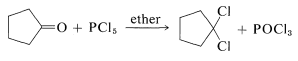This reaction is useful in conjunction with $$E2$$ elimination to prepare alkenyl halides, allenes, and alkynes. Cycloalkenyl halides are easily prepared, but because of angle strain the cycloalkynes and cycloallenes with fewer than eight atoms in the ring cannot be isolated (see Section 12-7):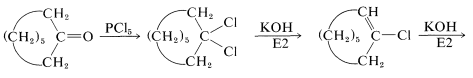Replacement of a carbonyl group by gem-fluorines$$^3$$ can be achieved with molybdenum hexafluoride or sulfur tetrafluoride. Sulfur tetrafluoride converts carboxyl functions to trifluoromethyl groups: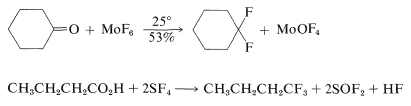## Hydride as a Nucleophile. Reduction of Carbonyl Compounds

### Metal and Boron Hydrides

In recent years, inorganic hydrides such as lithium aluminum hydride, $$\ce{LiAlH_4}$$, and sodium borohydride, $$\ce{NaBH_4}$$, have become extremely important as reducing agents of carbonyl compounds. These reagents have considerable utility, especially with sensitive and expensive carbonyl compounds. The reduction of cyclobutanone to cyclobutanol is a good example, and you will notice that the net reaction is the addition of hydrogen across the carbonyl double bond,$$\overset{ 2 \left[ \ce{H} \right]}{\longrightarrow}$$,With the metal hydrides, the key step is transfer of a hydride ion to the carbonyl carbon of the substance being reduced.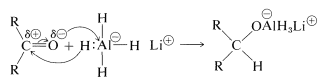The hydride transfer is analogous to the transfer of $$\ce{R}^\ominus$$ from organometallic compounds to carbonyl groups (Section 14-12A).

Lithium aluminum hydride is best handled like a Grignard reagent, because it is soluble in ether and is sensitive to both oxygen and moisture. (Lithium hydride is insoluble in organic solvents and is not an effective reducing agent for organic compounds.) All four hydrogens on aluminum can be utilized: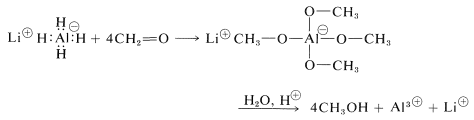The reaction products must be decomposed with water and acid as with the Grignard complexes. Any excess lithium aluminum hydride is decomposed by water and an acid with evolution of hydrogen:

$\ce{LiAlH_4} + 2 \ce{H_2SO_4} \rightarrow \frac{1}{2} \ce{Li_2SO_4} + \frac{1}{2} \ce{Al_2(SO_4)_3} + 4 \ce{H_2}$

Lithium aluminum hydride usually reduces carbonyl groups without affecting carbon-carbon double bonds. It is, in addition, a good reducing agent for carbonyl groups of carboxylic acids, esters, and other acid derivatives, as will be described in Chapter 18.

Sodium borohydride is a milder reducing agent than lithium aluminum hydride and will reduce aldehydes and ketones, but not acids or esters. It reacts sufficiently slowly with water in neutral or alkaline solution that reductions which are reasonably rapid can be carried out in water solution without appreciable hydrolysis of the reagent:

$\ce{NaBH_4} + 4 \ce{CH_2=O} + 3 \ce{H_2O} \rightarrow 4 \ce{CH_3OH} + \ce{NaOB(OH)_2}$

Borane (as $$\ce{BH_3}$$ in tetrahydrofuran or dimethyl sulfide) is an even milder reducing agent than $$\ce{BH_4^+}$$ for the carbonyl group of aldehydes and ketones. This difference in reactivity can be used to advantage when selective reduction is necessary. For example, borohydride reduces a ketone carbonyl more rapidly than a carbon-carbon double bond, whereas borane reduces the carbon-carbon double bond more rapidly than carbonyl:A useful comparison of the reactivities of boranes and metal hydrides toward various types of multiple bonds is given in Table 16-6.

Table 16-6: Comparison of Products and Reactivities of Functional Groups for Reduction with Borane and Metal Hydrides$$^{a,b}$$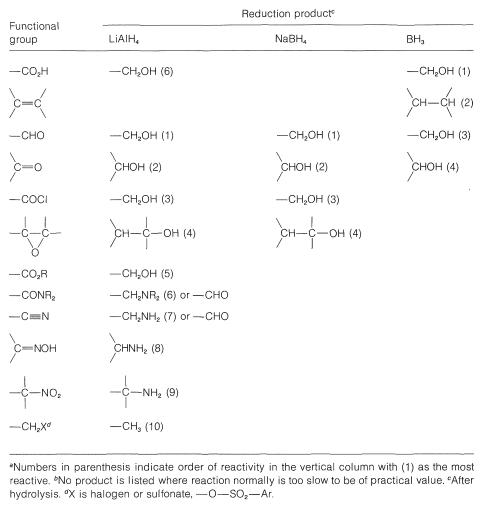### The Cannizzaro Reaction

A characteristic reaction of aldehydes without $$\alpha$$ hydrogens is the self oxidation-reduction they undergo in the presence of strong base. With methanal as an example,If the aldehyde has $$\alpha$$ hydrogens, other reactions usually occur more rapidly.

The mechanism of this reaction, usually called the Cannizzaro reaction,$$^4$$ combines many features of other processes studied in this chapter. The first step is reversible addition of hydroxide ion to the carbonyl group: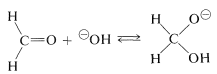A hydrogen can be transferred as hydride ion to methanal from the hydroxyalkoxide ion, thereby reducing the methanal to methanol:### Reduction with Aluminum Alkoxides

Hydride transfer similar to that of the Cannizzaro reaction also may be achieved from a $$\ce{C-H}$$ grouping in an alkoxide ion corresponding to a primary or secondary, but not a tertiary, alcohol. This is expected to be a reversible reaction, because the products are another alkoxide and another carbonyl compound:To utilize this equilibrium process as a practical reduction method requires rather special conditions. It is preferable to use an aluminum alkoxide, $$\ce{Al(OR)_3}$$, rather than a sodium alkoxide, $$\overset{\oplus}{\ce{Na}} \overset{\ominus}{\ce{O}} \ce{R}$$, to ensure that the reaction mixture is not too strongly basic. (Carbonyl compounds, particularly aldehydes, are sensitive to strong bases.) The overall reaction may be written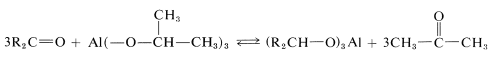for which the alkoxide is derived from 2-propanol. The advantage of this method is that the reaction can be driven essentially to completion by distilling out the 2-propanone as it is formed. The reduction product subsequently can be obtained by acid hydrolysis of the aluminum alkoxide: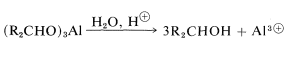### Biological Reactions

These have been discussed already in the context of the reverse reactions - oxidation of alcohols (Section 15-6C).

$$^2$$Pronounced variously as ill/id, yill/id, ill/ide, yill/ide. The dipolar structures usually written for ylides are an oversimplified representation of the bonding in these substances.

$$^3$$Gem is an abbreviation for geminal (twinned) and is a common conversational designation for arrangements having two identical substituents on one carbon.

$$^4$$Named after its discoverer, the same Cannizzaro who, in 1860, made an enormous contribution to the problem of obtaining self-consistent atomic weights (Section 1-1).